﻿

### Codimension two product submanifolds with non-negative curvature

#### Abstract

We prove that if(Error rendering LaTeX formula) is an isometric immersion of a complete, non-compact Riemannian manifold M which is a product of non-negatively curved manifolds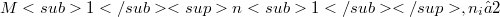$M1n1, n_i≥ 2$,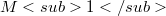$M1$ non-flat and irreducible, then either f is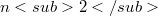$n2$-cylindrical; or f is a product of hypersurface immersions with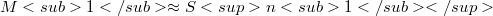$M1 \approx Sn1$ or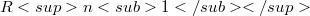$Rn1$; or f is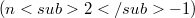$(n2-1)$-cylindrical with$M1\approx Sn1$ or$RP2$ when$M1$ is compact, and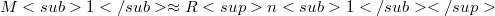$M1\approx Rn1$ when$M1$ is non-compact.

DOI Code: 10.1285/i15900932v9n1p89

Full Text: PDF